x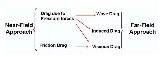Aerodynamic dragEncyclopedia
Aerodynamic drag is the fluid drag force
Drag (physics)
In fluid dynamics, drag refers to forces which act on a solid object in the direction of the relative fluid flow velocity...

that acts on any moving solid body in the direction of the fluid freestream
Freestream
The freestream is the air far upstream of an aerodynamic body, that is, before the body has a chance to deflect, slow down or compress the air. Freestream conditions are usually denoted with a \infty symbol, e.g. V_\infty, meaning the freestream velocity...

flow. From the body's perspective (near-field approach), the drag comes from forces due to pressure distributions over the body surface, symbolized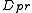, and forces due to skin friction, which is a result of viscosity, denoted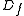. Alternatively, calculated from the flowfield perspective (far-field approach), the drag force comes from three natural phenomena: shock waves
Shock Waves
Shock Waves, , is a 1977 horror movie written and directed by Ken Wiederhorn...

, vortex sheet and viscosity
Viscosity
Viscosity is a measure of the resistance of a fluid which is being deformed by either shear or tensile stress. In everyday terms , viscosity is "thickness" or "internal friction". Thus, water is "thin", having a lower viscosity, while honey is "thick", having a higher viscosity...

.

## Introduction

The pressure distribution over the body surface exerts normal forces which, summed and projected into the freestream direction, represent the drag force due to pressure. The nature of these normal forces combines shock wave effects, vortex system generation effects and wake viscous mechanisms all together.

When the viscosity effect over the pressure distribution is considered separately, the remaining drag force is called pressure (or form) drag. In the absence of viscosity, the pressure forces on the vehicle cancel each other and, hence, the drag is zero. Pressure drag is the dominant component in the case of vehicles with regions of separated flow, in which the pressure recovery is fairly ineffective.

The friction drag force, which is a tangential force on the aircraft surface, depends substantially on boundary layer configuration and viscosity. The calculated friction dragutilizes the x-projection of the viscous stress tensor evaluated on each discretized body surface.

The sum of friction drag and pressure (form) drag is called viscous drag. This drag component takes into account the influence of viscosity. In a thermodynamic perspective, viscous effects represent irreversible phenomena and, therefore, they create entropy. The calculated viscous drag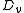use entropy changes to accurately predict the drag force.

When the airplane produces lift, another drag component comes in. Induced drag, symbolized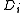, comes about due to a modification on the pressure distribution due to the trailing vortex system that accompanies the lift production. Induced drag tends to be the most important component for airplanes during take-off or landing flight. Other drag component, namely wave drag,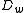, comes about from shock waves in transonic and supersonic flight speeds. The shock waves induce changes in the boundary layer and pressure distribution
over the body surface. It is worth noting that not only viscous effects but also shock waves induce irreversible phenomena and, as a consequence, they can be measured through entropy changes along the domain as well. The figure below is a summary of the various aspects previously discussed.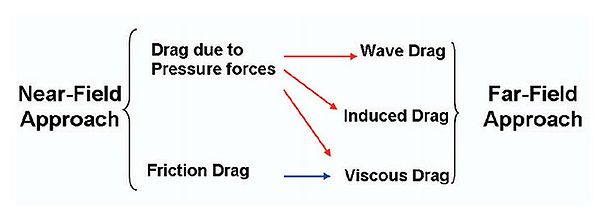## Theoretical aspects of far-field/near-field balance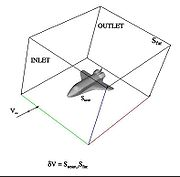The drag force calculation can be performed using the integral of force balance in the freestream direction as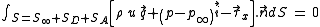which surrounds the body represents the union of two unconnected surfaces,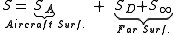where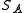is the airplane surface,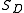is the outlet surface and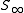represents both the lateral and inlet surfaces. In general, the far-field control volume is located in the boundaries of the domain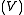and its choice is user-defined. In Subsection \ref{sGF}, further considerations concerning to the correct selection of the far-field boundary are given, allowing for desired flow characteristics.

Equation (\ref{ta1}) can be decomposed into two surface integrals, yielding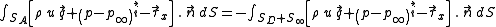The right-hand side integral in Eq.\ (\ref{ta2}) represents the reaction forces of the airplane. The left-hand side integral in Eq.\
(\ref{ta2}) represents the total force exerted by the fluid. Mathematically, these two integrals are equivalent. However, the numerical integration of these terms will hardly lead to the same result, because the solution is approximated. In the terminology of Computational Fluid Dynamics (CFD), when the integration is performed using the left-hand side integral in Eq.\ (\ref{ta2}), the near-field method is employed. On the other hand, when the integration of the right-hand side in Eq. (\ref{ta2}) is computed, the far-field method is considered.

The drag force balance is assured mathematically by Eq.\ (\ref{ta2}), that is, the resultant drag force evaluated using the near-field approach must be equal to the drag force extracted by the far-field approach. The correct drag breakdown considered in this work is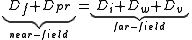• Aerodynamic force
• Drag (physics)
Drag (physics)
In fluid dynamics, drag refers to forces which act on a solid object in the direction of the relative fluid flow velocity...

• Drag equation
Drag equation
In fluid dynamics, the drag equation is a practical formula used to calculate the force of drag experienced by an object due to movement through a fully enclosing fluid....

• Drag coefficient
Drag coefficient
In fluid dynamics, the drag coefficient is a dimensionless quantity that is used to quantify the drag or resistance of an object in a fluid environment such as air or water. It is used in the drag equation, where a lower drag coefficient indicates the object will have less aerodynamic or...

• Lift (force)
Lift (force)
A fluid flowing past the surface of a body exerts a surface force on it. Lift is the component of this force that is perpendicular to the oncoming flow direction. It contrasts with the drag force, which is the component of the surface force parallel to the flow direction...